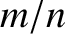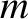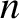# Introduction

As was explained in Section 5.1, when describing the response of a tokamak plasma to a tearing mode perturbation, the plasma can be divided into two regions . In the outer region, which comprises most of the plasma, the tearing perturbation is governed by the equations of marginally-stable ideal-MHD. However, these equations become singular at the rational magnetic flux-surface where the safety-factor (see Section 3.2) takes the value. Here,andare the poloidal and toroidal mode numbers, respectively, of the tearing mode. A radially thin region forms around the rational magnetic flux-surface, and constitutes the inner region. As was discussed in Section 5.16, the response of the plasma in the inner region to a rotating tearing perturbation in the outer region is accurately described by linear theory only when the width of the magnetic island chain that develops at the rational surface is less than the linear layer width. In the opposite limit, in which the island width exceeds the layer width, a nonlinear approach is required. The aim of this chapter is to employ the reduced drift-MHD model derived in Chapter 4 to determine the nonlinear response of the plasma in the inner region to a rotating tearing perturbation in the outer region.